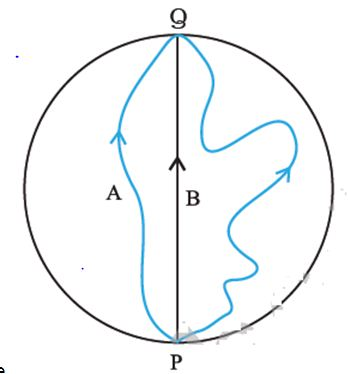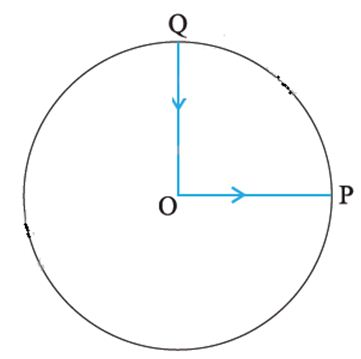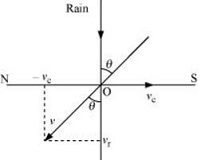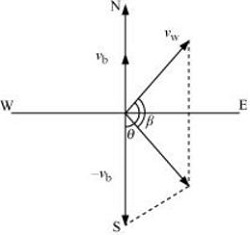# Topic: Motion in A Plane (Test 3)

Topic: Motion in A Plane
Q.1
Which of the following algebraic operations on vectors not permissible?
A. adding a scalar component of the vector to the same vector
B. multiplying any vector by any scalar,
C. multiplying the sum of vectors A and B by a scalar
Explaination / Solution:

Although vectors and scalars represent different types of physical quantities, it is sometimes necessary for them to interact. While adding a scalar to a vector is impossible because of their different dimensions in space, it is possible to multiply a vector by a scalar.

Workspace
Report
Q.2
Which of the following statements false?
A. the total path length is always equal to the magnitude of the displacement vector of a particle
B. the magnitude of a vector is always a scalar
C. Three vectors not lying in a plane can never add up to give a null vector.
D. the average speed of a particle (defined as total path length divided by the time taken to cover the path) is either greater or equal to the magnitude of average velocity of the particle over the same interval of time,
Explaination / Solution:

the average speed of a particle (defined as total path length divided by the time taken to cover the path) is either greater or equal to the magnitude of average velocity of the particle over the same interval of time,

Workspace
Report
Q.3
Given vectors a, b, c, d and a + b + c + d = 0, which of the following statements not correct?
A. The magnitude of (a + c) equals the magnitude of ( b + d)
B. b + c must lie in the plane of a and d if a and d are not collinear, and in the line of a and d, if they are collinear
C. a, b, c and d must each be a null vector
D. The magnitude of a can never be greater than the sum of the magnitudes of b, c, and d
Explaination / Solution:

In order to make vectors a + b + c + d = 0, it is not necessary to have all the four given vectors to be null vectors. There are many other combinations which can give the sum zero. (b) Correct a + b + c + d = 0 a + c = – (b + d) Taking modulus on both the sides, we get: | a + c | = | –(b + d)| = | b + d | Hence, the magnitude of (a + c) is the same as the magnitude of (b + d). (c) Correct a + b + c + d = 0 a = – (b + c + d) Taking modulus both sides, we get: | a | = | b + c + d | | a | ≤ | a | + | b | + | c | ..... (i) Equation (i) shows that the magnitude of a is equal to or less than the sum of the magnitudes of b, c, and d. Hence, the magnitude of vector a can never be greater than the sum of the magnitudes of b, c, and d. (d) Correct For a + b + c + d = 0 a + (b + c) + d = 0 The resultant sum of the three vectors a, (b + c), and d can be zero only if (b + c) lie in a plane containing a and d, assuming that these three vectors are represented by the three sides of a triangle. If a and d are collinear, then it implies that the vector (b + c) is in the line of a and d. This implication holds only then the vector sum of all the vectors will be zero.

Workspace
Report
Q.4
Three girls skating on a circular ice ground of radius 200 m start from a point P on the edge of the ground and reach a point Q diametrically opposite to P following different paths as shown in Figure. What is the magnitude of the displacement vector for each ? For which girl is this equal to the actual length of path skate ?A. 300 m for each; C
B. 200 m for each; A
C. 200 m for each; B
D. 400 m for each; B
Explaination / Solution:

(i) PQ = diameter = displacement for each girl = 2r = 2 x 200 = 400 m Since, displacement vector does not depend upon the actual path length and it is the shortest distance between initial and final position, so in the case of each girl the displacement is 400 m. (ii)This is equal to the actual length of the path skated by girl B.

Workspace
Report
Q.5
A cyclist starts from the centre O of a circular park of radius 1 km, reaches the edge P of the park, then cycles along the circumference anticlockwise from P to Q, and returns to the centre along QO as shown in Figure. If the round trip takes 10 min, what is the (a) net displacement, (b) average velocity, and (c) average speed of the cyclist ?A. 0, 0, 15.4 km/hr
B. 0, 0, 27.4 km/hr
C. 0, 0, 11.4 km/hr
D. 0, 0, 21.4 km/hr
Explaination / Solution:

(a) displacement is shortest distance b/w initial point and final point, as he returns back to origin after starting from origin, the displacement is zero.

(b) Average velocity =

As displacement is zero, so average velocity is also eqaul to zero.

(c) Radius r = 1 km

distance covered = OP+PQ+QO

$\frac{25}{7}$km

Time taken = 10 minutes = $\frac{1}{6}$hour

Average speed  $=$

21.4 km/h

Workspace
Report
Q.6
A passenger arriving in a new town wishes to go from the station to a hotel located 10 km away on a straight road from the station. A dishonest cabman takes him along a circuitous path 23 km long and reaches the hotel in 28 min. What is (a) the average speed of the taxi, (b) the magnitude of average velocity?
A. 47.3 km/hr, 23.4 km/hr
B. 48.3 km/hr, 22.4 km/hr
C. 46.3 km/hr, 24.4 km/hr
D. 49.3 km/hr, 21.4 km/hr
Explaination / Solution:

(a) Total distance travelled = 23 km
Total time taken = 28 min =  h
∴  Average speed of the taxi =

(b) Distance between the hotel and the station = 10 km = Displacement of the car
∴ Average velocity =

Workspace
Report
Q.7
Rain is falling vertically with a speed of 30 m . A woman rides a bicycle with a speed of 10 m  in the north to south direction. What is the direction in which she should hold her umbrella?
A. about  with the vertical, towards the north
B. about  with the vertical, towards the south
C. about  with the vertical, towards the south
D. about  with the vertical, towards the north
Explaination / Solution:

The described situation is shown in the given figure.Here,

vc = Velocity of the cyclist
vr = Velocity of falling rain

In order to protect herself from the rain, the woman must hold her umbrella in the direction of the relative velocity (v) of the rain with respect to the woman.
v = vr + (-vc)
= 30 + (-10) = 20 m/s
tan θ =
θ = tan-1

Workspace
Report
Q.8
A man can swim with a speed of 4.0 km/h in still water. How long does he take to cross a river 1.0 km wide if the river flows steadily at 3.0 km/h and he makes his strokes normal to the river current? How far down the river does he go when he reaches the other bank?
A. 15 min, 750 m
B. 16 min, 650 m
C. 19 min, 500 m
D. 13 min, 700 m
Explaination / Solution:

Speed of the man, vm = 4 km/h
Width of the river = 1 km
Time taken to cross the river =

Speed of the river, vr = 3 km/h
Distance covered with flow of the river = vr × t

= 750 m

Workspace
Report
Q.9
In a harbor, wind is blowing at the speed of 72 km/h and the flag on the mast of a boat anchored in the harbor flutters along the N-E direction. If the boat starts moving at a speed of 51 km/h to the north, what is the direction of the flag on the mast of the boat?
A. approximately north
B. approximately north-east
C. approximately south
D. approximately east
Explaination / Solution:

The flag is fluttering in the north-east direction. It shows that the wind is blowing toward the north-east direction. When the ship begins sailing toward the north, the flag will move along the direction of the relative velocity (vwb) of the wind with respect to the boat.The angle between vw and (–vb) = 90° + 45°

tan β =

Substituting and solving we get,

tan β =

∴ β = tan-1(1.0038) = 45.110

Angle with respect to the east direction = 45.11° – 45° = 0.11°

Hence, the flag will flutter approximately due east.

Workspace
Report
Q.10
The ceiling of a long hall is 25 m high. What is the maximum horizontal distance that a ball thrown with a speed of 40 m can go without hitting the ceiling of the hall?
A. 165 m
B. 150.5 m
C. 25 m
D. 120 m
Explaination / Solution:

Maximum height, h = 25 m
In projectile motion, the maximum height reached by a body projected at an angle θ, is given by the relation:
h =
=> 25 =
=  sin2θ = 0.30625
sin θ = 0.5534
∴ θ = sin–1(0.5534) = 33.60°
Horizontal range, R =

=  150.5 m

Workspace
Report